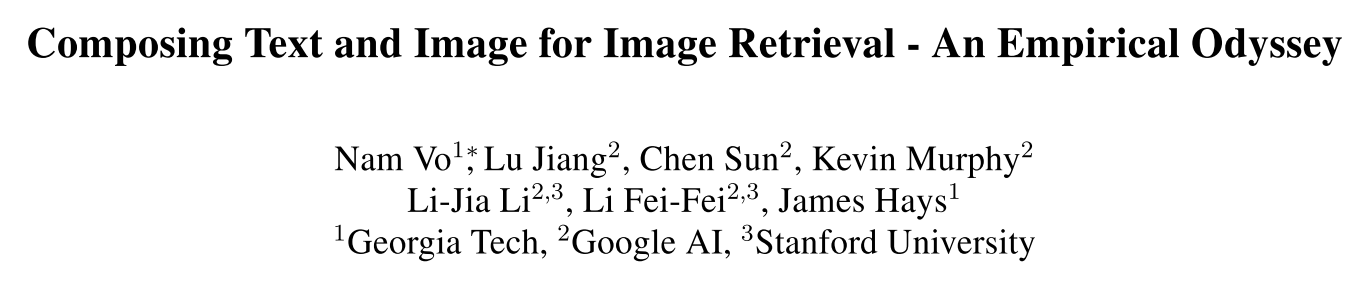## 论文信息

• 作者：Nam S. Vo, Lu Jiang, Chen Sun, Kevin Murphy, Li-Jia Li, Li Fei-Fei, James Hays
• 出处：CVPR 2019
• 关键词：Image retrieval, multimodal, metric learning, feature composition
• 论文链接
• 其他资料：

## 内容简记

### 背景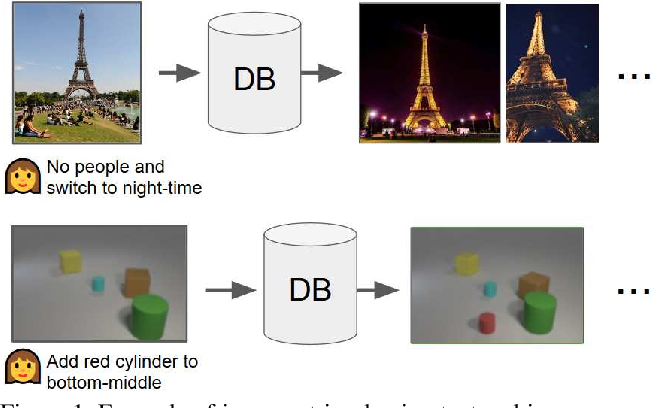• 拼接输入 MLP；
• Show and Tell ：用一个 LSTM 同时编码图像和文本，先输入图像特征，后输入文本单词。LSTM 的最后一个状态作为表征；
• Attribute as Operator ：将文本编码为转移矩阵，并应用到图像特征上得到组合特征；
• Parameter hashing ：已被编码的文本特征被散列为变换矩阵，用于替代 CNN 网络中的全连接层，以得到组合特征；
• Relationship ：首先使用 CNN 提取图像的 2d 特征图（feature map），然后创建一组关系特征，每个特征是 2d 特征图中的 2 个局部特征与文本特征的拼接。这组特征被传入一个 MLP 中并将输出平均化以得到组合特征；
• FiLM ：文本特征被用于预测调制特征（modulation features） $\gamma^{i}, \beta^{i} \in \mathbb{R}^{C}$，其中 $i$ 为层的索引（index），$C$ 为特征的数量。之后执行图像特征的仿射变换：$\phi_{x t}^{i}=\gamma^{i} \cdot \phi_{x}^{i}+\beta^{i}$。

• TIRG 使用非线性变换（而非 FiLM 中的线性变换），具有更多可学习参数，使得能够对图像进行较为复杂的修改；
• TIRG 只修改单层的 CNN。修改尽可能少的层有助于确保修改的特征处于目标图像的相同空间中。

### 方法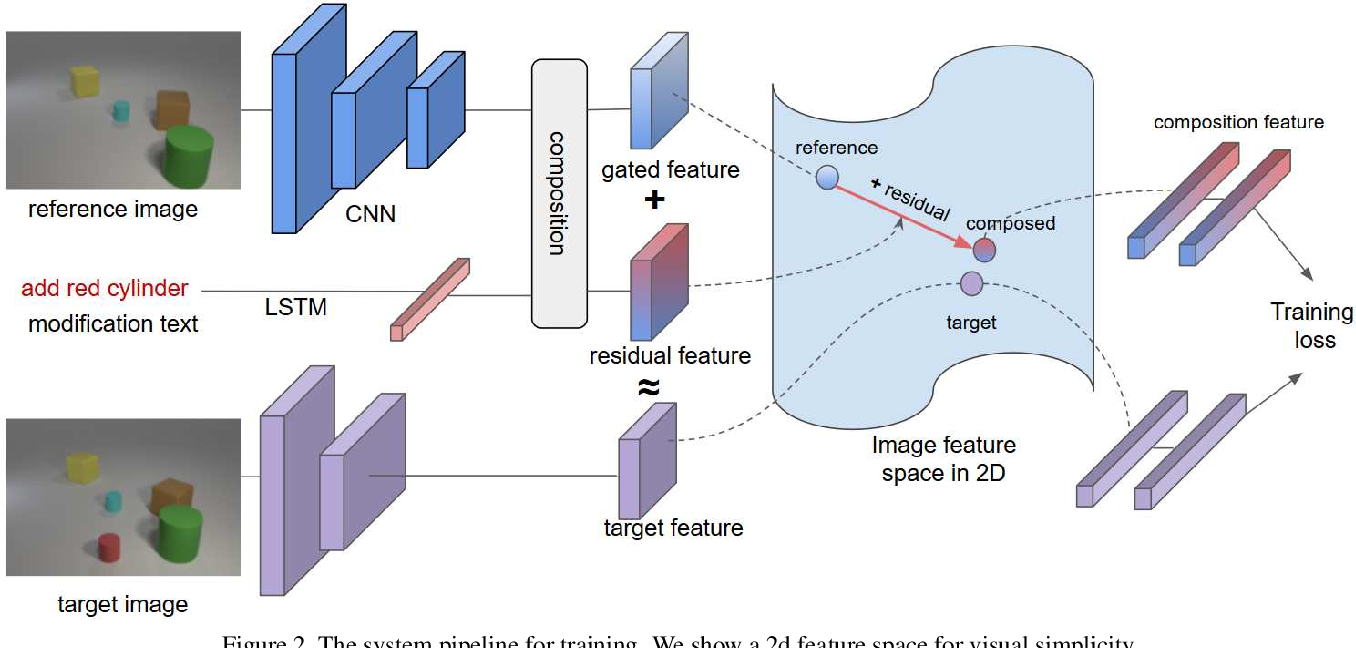TIRG 的公式为：

$$\phi_{x t}^{r g}=w_{g} f_{\mathrm{gate}}\left(\phi_{x}, \phi_{t}\right)+w_{r} f_{\mathrm{res}}\left(\phi_{x}, \phi_{t}\right)$$

$$f_{\text {gate }}\left(\phi_{x}, \phi_{t}\right)=\sigma\left(W_{g 2} * {RELU}\left(W_{g 1} *\left[\phi_{x}, \phi_{t}\right]\right) \odot \phi_{x}\right.$$

$$f_{\text {res }}\left(\phi_{x}, \phi_{t}\right)=W_{r 2} * {RELU}\left(W_{r 1} *\left(\left[\phi_{x}, \phi_{t}\right]\right)\right)$$

TIRG 的直观理解是我们只想根据文本特征“修改”图像特征，而不是创建一个完全不同的特征空间。门控机制被设计成保留某些与文本修改无关的查询图像特征。

#### 训练方法

$$L=\frac{-1}{M B} \sum_{i=1}^{B} \sum_{m=1}^{M} \log \left\{\frac{\exp \left\{\kappa\left(\psi_{i}, \phi_{i}^{+}\right)\right\}}{\sum_{\phi_{j} \in \mathcal{N}_{i}^{m}} \exp \left\{\kappa\left(\psi_{i}, \phi_{j}\right)\right\}}\right\}$$

### 实验

#### 对比实验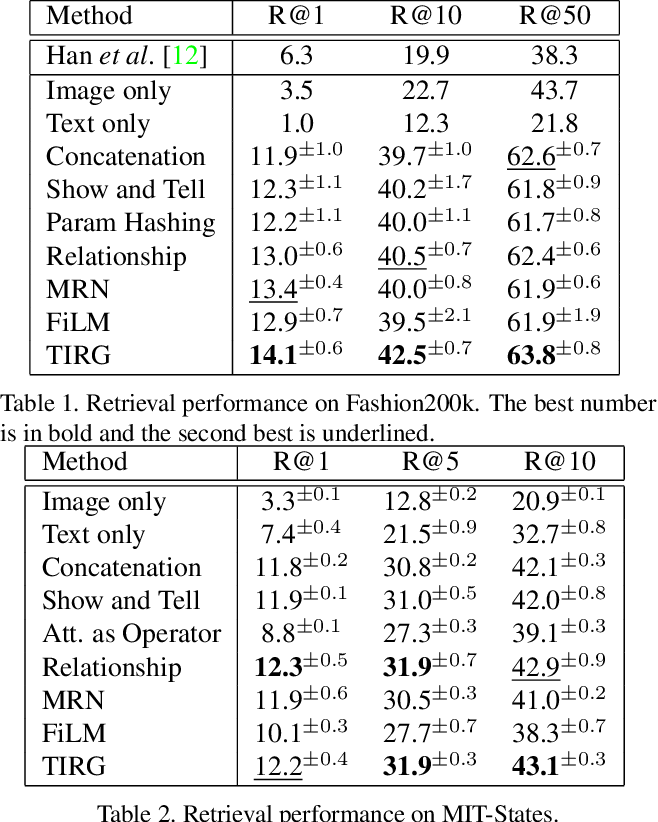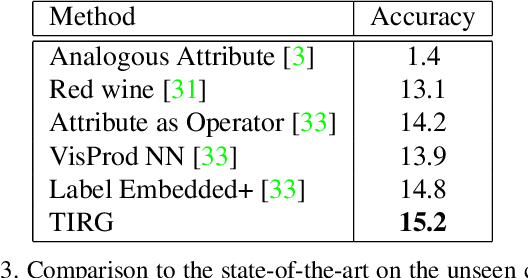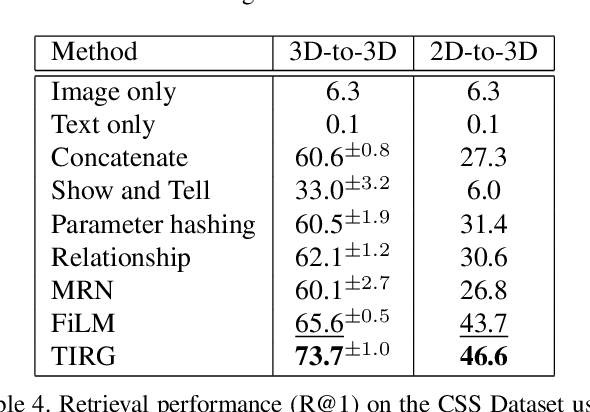#### 从组合特征中重建图像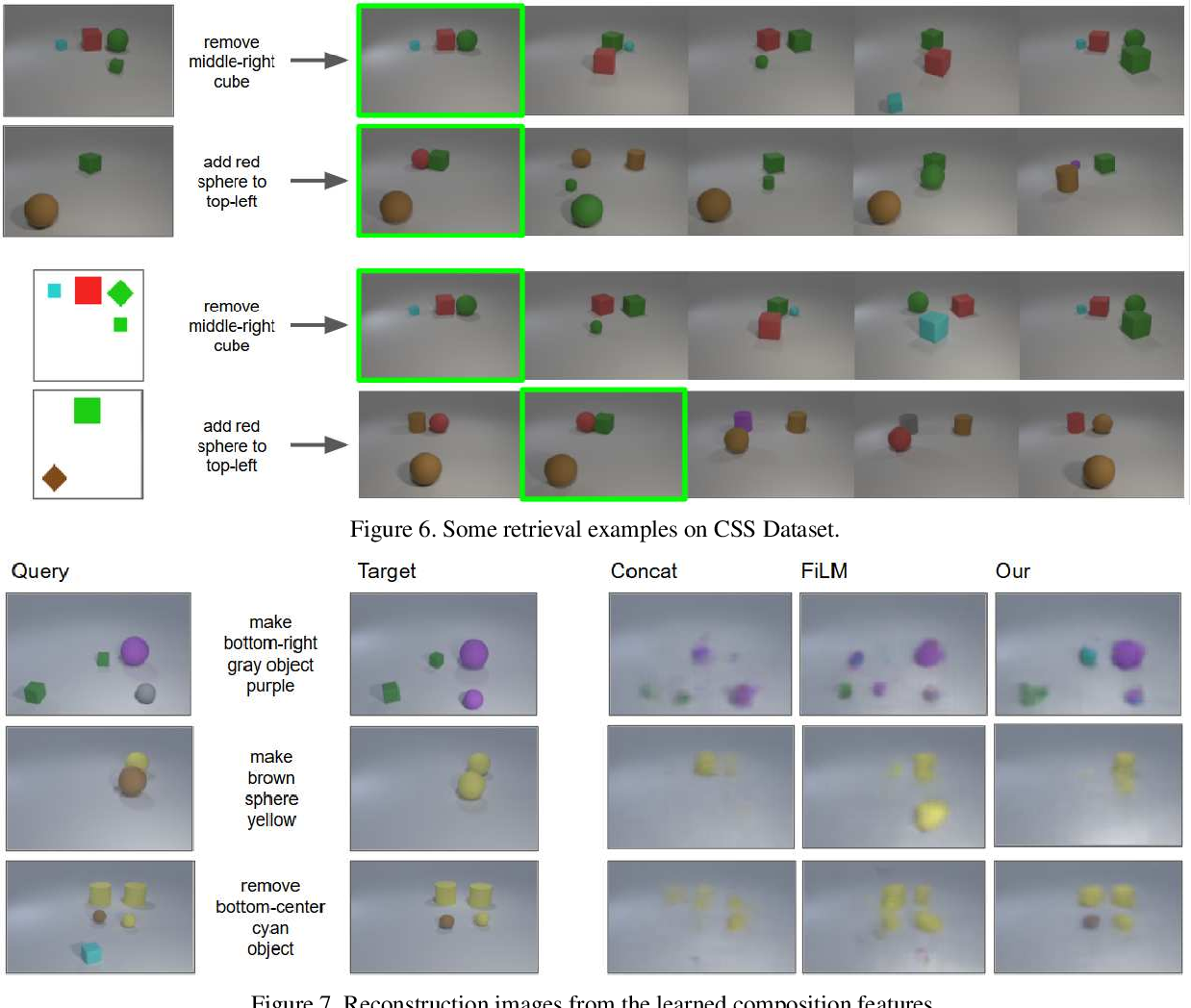#### 消融实验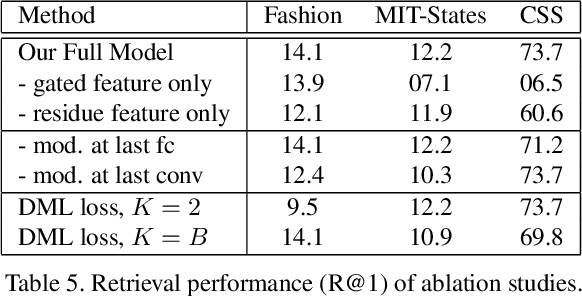1. 去除残差连接和门控机制都会使模型表现显著变差；
2. TIRG 的特征修改可发生在最后一个卷积层或最后一个全连接层。实验表明，对于 Fashion200k 和 MIT-States 数据集在最后一个全连接层进行特征修改，以及对于 CSS 数据集在最后一个卷积层进行特征修改，效果更好。作者认为这是因为在 CSS 数据集上的修改更加空间局部化，而在其他两个数据集上的修改更加全局化；
3. 设置训练时的每次采样的样本数量为 2（即损失变为 soft triplet loss）或 B（即实验中原本的设置）。当采样大小为 2 时，会发现对于 Fashion200k 这样较大的数据集，网络会欠拟合；而当采样大小为 B 时，网络对 Fashion200k 数据集拟合更好并得到更好的结果，但是对于其他两个数据集训练变得不稳定。

CNN：

LSTM：

## 参考论文

•  A simple neural network module for relational reasoning, NIPS 2017.
•  Image question answering using convolutional neural network with dynamic parameter prediction, CVPR 2016.
•  Show and tell: A neural image caption generator, CVPR 2015.
•  Attributes as operators, 2018.
•  Film: Visual reasoning with a general conditioning layer, 2018.
•  Automatic spatially-aware fashion concept discovery, ICCV 2017.
•  Discovering states and transformations in image collections, CVPR 2015.